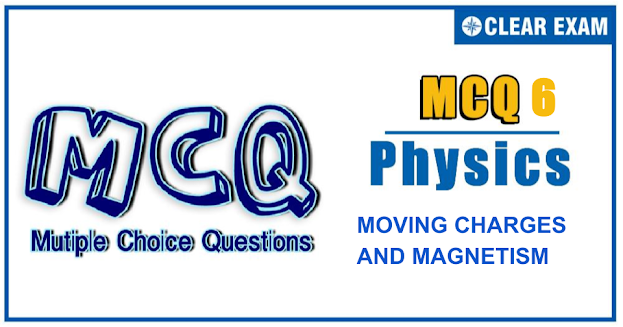## [LATEST]\$type=sticky\$show=home\$rm=0\$va=0\$count=4\$va=0

JEE Advanced Physics Syllabus can be referred by the IIT aspirants to get a detailed list of all topics that are important in cracking the entrance examination. JEE Advanced syllabus for Physics has been designed in such a way that it offers very practical and application-based learning to further make it easier for students to understand every concept or topic by correlating it with day-to-day experiences. In comparison to the other two subjects, the syllabus of JEE Advanced for physics is developed in such a way so as to test the deep understanding and application of concepts.

Q1.  Two identical particles having the same mass m and charges +q and -q separated by a distance d enter a uniform magnetic field B directed perpendicular to paper inwards with speeds v_1 and v_2 as shown in Figure. The particles will not collide if
•  d>m/Bq (v_1+v_2)
•  d<m/Bq (v_1+v_2)
•  d>2m/Bq (v_1+v_2)
•  v_1=v_2
Q2.If a changed particle of charge to mass ratio (q/m)=α enters in a magnetic field of strength B at a speed v=(2αd)(B), then
•  Angle subtended by the path of charged particle in magnetic field at the centre of circular path is 2π
•  The charge will move on a circular path and then will come out from magnetic field at some distance from the point of insertion
•  The time for which particle will be in the magnetic field is 2π/αB
•  Angle subtended by the path of charged particle in magnetic field at the centre of circular path is π/2
Q3.  The figure shows certain wire segments joined together to form a coplanar loop. The loop is placed in a perpendicular magnetic field in the direction going into the plane of the figure. The magnitude of the field increases with time. I_1 and I_2 are the currents in segment ab and cd. Then,

•  I_1>I_2
•  >I_1<I_2
•  <I_1 is in the direction ba and I_2 is in the direction cd
•  I_1 is in the direction ab and I_2 is in the direction dc
Solution

Q4. A conducting circular loop of radius r carries a constant current i. It is placed in a uniform magnetic field B_0 perpendicular to the plane of the loop. The magnetic force acting on the loop is
•  irB_0
•  2πirB_0
•  Zero
•  πirB_0
Solution
Q5. A wire of cross-sectional area A forms three sides of a square and is free to rotate about axis OO'. if the   structure is deflected by an angle θ from the vertical when current i is passed through it in a magnetic filed B acting vertically upward and density of the wire is ρ, then the value of θ is given by

•  2Aρg/iB=cot⁡θ
•  2Aρg/iB=tan⁡θ
•  Aρg/iB=sin⁡θ
•  Aρg/2iB=cos⁡θ
Q6. A long, straight, hollow conductor (tube) carrying a current has two sections A and C of unequal cross sections joined by a conical section B. 1, 2 and 3 are points on a line parallel to the axis of the conductor. The magnetic fileds at 1,2 and 3 have magnitudes B_1,B_2 and B_3.Then,
•  B_1=B_2=B_3
•  B_1=B_2≠B_3
• B_1<B_2<B_3
•  B_2 cannot be found unless the dimensions of the section B are known
Q7.The rigid conducting thin wire frame carries an electric current I and this frame is inside a uniform magnetic field B ⃗ as shown in Figure. Then,

•  The net magnetic force on the frame is zero but the torque is not zero
•  The net magnetic force on the frame and the torque due to magnetic field are both zero
•  The net magnetic force on the frame is not zero and the torque is also not zero
•  None of these
Q8.An insulating rod of length l carries a charge q distributed uniformly on it. The rod is pivoted at its mid-point and is rotated at a frequency f about a fixed axis perpendicular to the rod and passing through the pivot. The magnetic moment of the rod system is

•  1/12 πqfl^2
•  Tπqfl^2
•  1/6 πqfl^2
•  1/3 πqfl^2
Q9.A point charge is moving in clockwise direction in a circle with constant speed. Consider the magnetic field produced by the charge at a fixed point P (not at the centre of circle) on the axis of the circle. Then,
•  It is constant in magnitude only
•  It is constant in direction only
•  It is constant both in direction and magnitude
•  It is constant neither in magnitude nor in direction
Q10. A particle of specific charge α is projected from origin with velocity v =V_0 i ̂-V_0 k ̂ In a uniform magnetic field B =-B_0 k ̂. Find time dependence of velocity of the particle

•  v ((t))=v_0 cos⁡〖(αB_0 t)〗 i ̂+v_0 sin⁡〖(αB_0 t)〗 j ̂-v_0 k ̂
•  v ((t))=-v_0 cos⁡〖(αB_0 t)〗 i ̂+v_0 sin⁡〖(αB_0 t)〗 j ̂+v_0 k ̂
•  v ((t))=-v_0 cos⁡〖(αB_0 t)〗 i ̂+v_0 sin⁡〖(αB_0 t)〗 j ̂-v_0 k ̂
• v ((t))=v_0 cos⁡〖(αB_0 t)〗 i ̂+v_0 sin⁡〖(αB_0 t)〗 j ̂+v_0 k ̂
Solution#### Written by: AUTHORNAME

AUTHORDESCRIPTION## Want to know more

Please fill in the details below:

## Latest NEET Articles\$type=three\$c=3\$author=hide\$comment=hide\$rm=hide\$date=hide\$snippet=hide

Name

ltr
item
BEST NEET COACHING CENTER | BEST IIT JEE COACHING INSTITUTE | BEST NEET & IIT JEE COACHING: Moving Charges and Magnetism-Quiz-6
Moving Charges and Magnetism-Quiz-6
https://1.bp.blogspot.com/-iS8sC3Jg22U/YOfslzpTeLI/AAAAAAAAAM4/u4SeAwhVGAc26u0WaXu2SpWcD7dSa85LwCLcBGAsYHQ/w623-h329/Quiz%2BImage%2B20%2B%25281%2529.png
https://1.bp.blogspot.com/-iS8sC3Jg22U/YOfslzpTeLI/AAAAAAAAAM4/u4SeAwhVGAc26u0WaXu2SpWcD7dSa85LwCLcBGAsYHQ/s72-w623-c-h329/Quiz%2BImage%2B20%2B%25281%2529.png
BEST NEET COACHING CENTER | BEST IIT JEE COACHING INSTITUTE | BEST NEET & IIT JEE COACHING
https://www.cleariitmedical.com/2021/07/moving-charges-and-magnetism-quiz-6.html
https://www.cleariitmedical.com/
https://www.cleariitmedical.com/
https://www.cleariitmedical.com/2021/07/moving-charges-and-magnetism-quiz-6.html
true
7783647550433378923
UTF-8

STAY CONNECTED